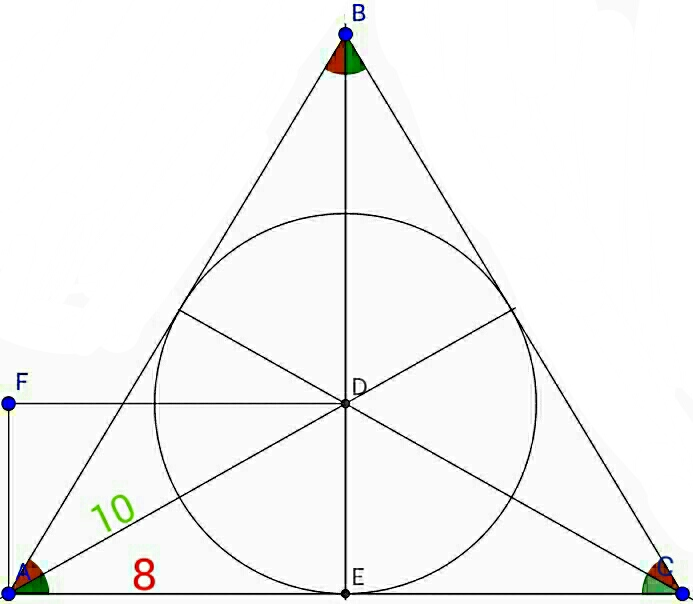# Circle gets cozy in triangle

Geometry Level 3In $\triangle{\text{ABC}}$, $\text{AD}$, $\text{BD}$ and $\text{CD}$ are the bisectors of $\angle{\text{BAC}}$, $\angle{\text{ABC}}$ and $\angle{\text{ACB}}$ respectively.
If $\text{AFDE}$ is a rectangle such that $\text{AD} = \text{10 cm}$ and $\text{AE} = \text{8 cm}$, find the area of the circle inscribed in $\triangle{\text{ABC}}$.

Round your answer( in ${\text{cm}}^{2}$) to the nearest integer.

×